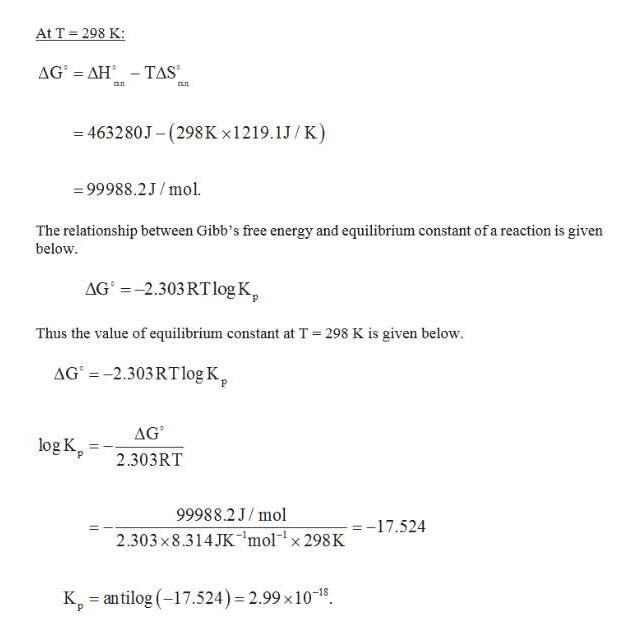# Carbon disulfide is a foul-smelling solvent that dissolvessulfur and other nonpolar substances. It can be made byheating sulfur in an atmosphere of methane:4 CH4(g) + S8(s) (equilibrium arrows) 4 CS2(g) +8 H2(g)Starting with the appropriate data in Appendix 4, calculatethe values of Kp for the reaction at 25°C and 500°C.(Appendix 4: CH4= -50720J, S8= 0, CS2= 65100J, H2=0

Question

Carbon disulfide is a foul-smelling solvent that dissolves
sulfur and other nonpolar substances. It can be made by
heating sulfur in an atmosphere of methane:
4 CH4(g) + S8(s) (equilibrium arrows) 4 CS2(g) +8 H2(g)

Starting with the appropriate data in Appendix 4, calculate
the values of Kp for the reaction at 25°C and 500°C.

(Appendix 4: CH4= -50720J, S8= 0, CS2= 65100J, H2=0

check_circleExpert Solution
Step 1

The balanced chemical reaction is given below.

Step 2

Heat of the reaction can be calculated as shown below.

Step 3

Suppose the change in enthalpy of the reaction is 1219.1 J/K. The chang...help_outlineImage Transcriptionclose298 K At T AG AH TAS an 463280J-(298K x1219.1J/ K) 99988.2J/mol. The relationship between Gibb's free energy and equilibrium constant of a reaction is given below AG'-2.303 RT log K Thus the value of equilibrium constant at T 298 K is given below. AG-2.303RTlog K AG log K 2.303RT 99988.2J/mol -17.524 2.303x8.314JK'molx 298K K antilog(17.524) 2.99 x1018. fullscreen

### Want to see the full answer?

See Solution

#### Want to see this answer and more?

Solutions are written by subject experts who are available 24/7. Questions are typically answered within 1 hour*

See Solution
*Response times may vary by subject and question
Tagged in

### Chemistry